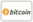Membrane Computing and Cryptographic Systems的封面

# Membrane Computing and Cryptographic Systems

LAP LAMBERT Academic Publishing (2015-10-13 )有获得代金券的资格
ISBN-13:

### 978-3-659-78026-4

ISBN-10:
365978026X
EAN:
9783659780264

Membrane computing is an area of computer science aiming to abstract computing ideas and models from the structure and the functioning of living cells. In short, it deals with distributed and parallel computing models, processing multiset with an essential role played by the communication among compartments where evolution rules and evolving objects are encapsulated into compartments delimited by membranes. RSA algorithm is the most widely used public-key cryptosystem. In practice, public-key encryption schemes are slower than symmetric-key counterparts due to using large numbers in power calculations . Since mathematical calculations need long time to perform encryption / decryption processes, In this book, two proposed designs of RSA using the membrane computing environment ideas are proposed. The first model depends on decreasing time of power calculations as it runs RSA algorithm as three parallel threads with all calculations which can be done parallel are executed at the same time.The second proposed design is depending on representing the parallel nature of membrane computing by using GPU model

https://www.lap-publishing.com/

168

2015-10-13

71.90 €

Membrane computing, RSA cryptography### 时事通讯